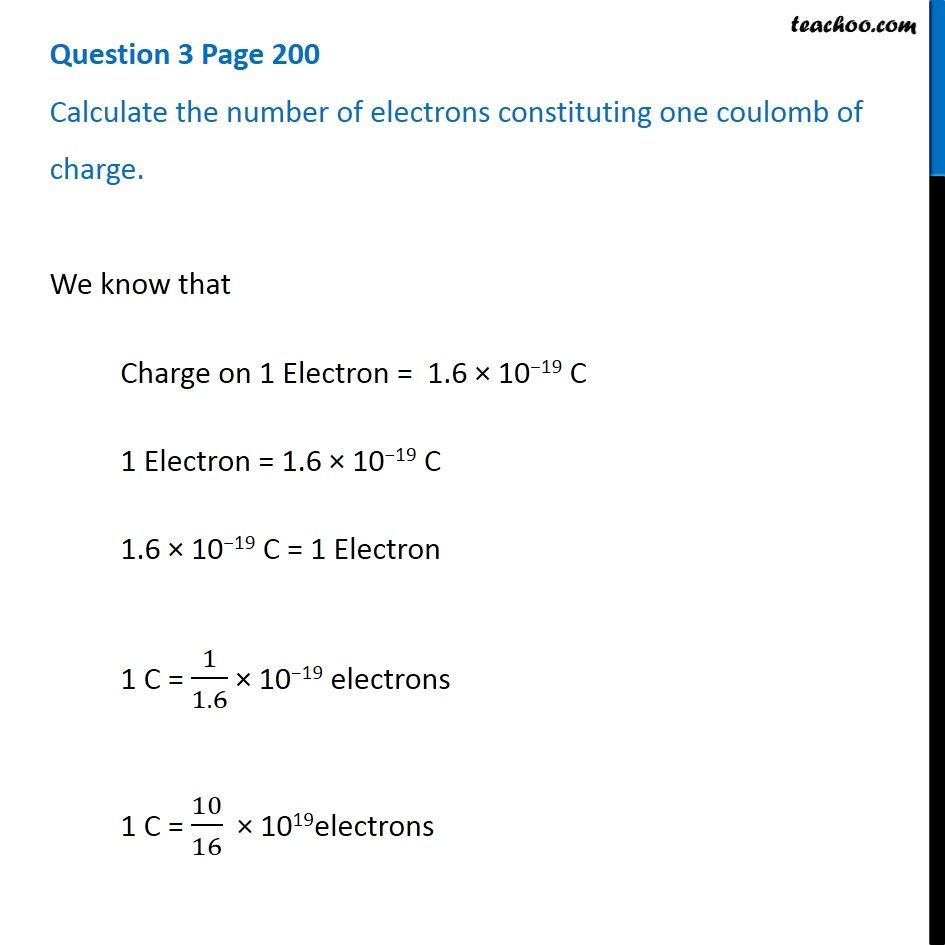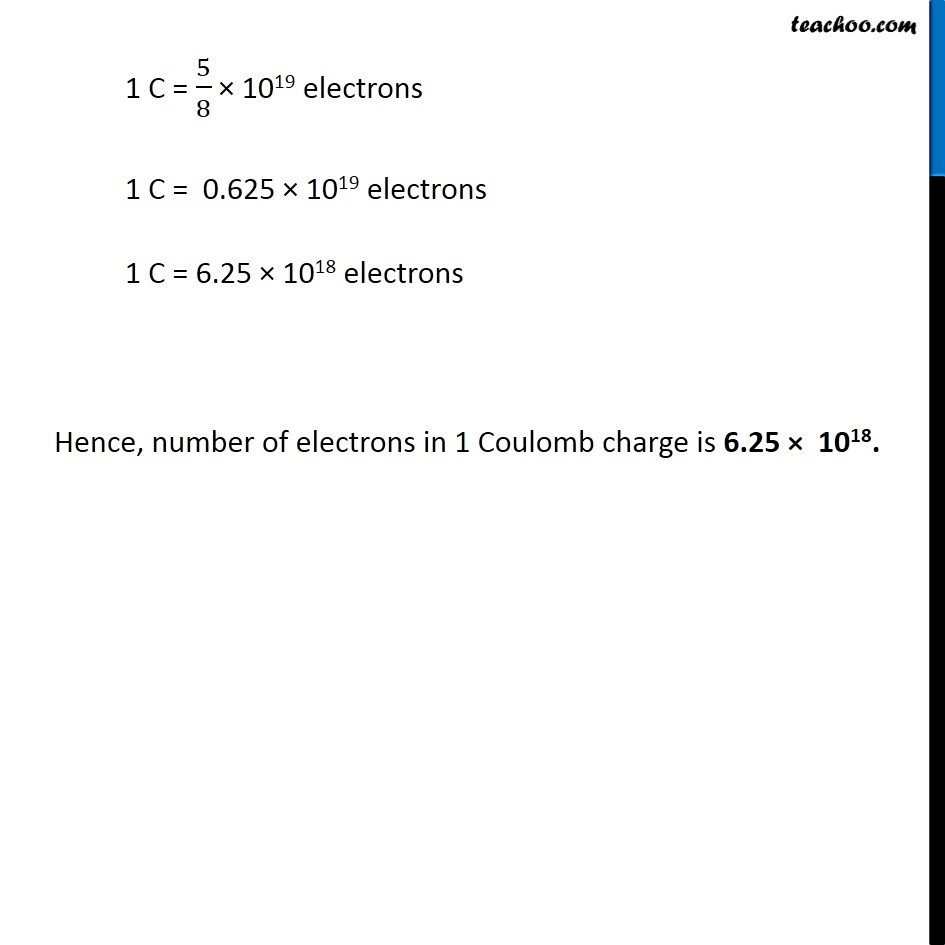Questions from Inside the chapter

Class 10
Chapter 12 Class 10 - Electricity

## Calculate the number of electrons constituting one coulomb of charge.

###googletag.cmd.push(function() { googletag.display('div-gpt-ad-1669298377854-0'); });Get live Maths 1-on-1 Classs - Class 6 to 12

### Transcript

Question 3 Page 200 Calculate the number of electrons constituting one coulomb of charge. We know that Charge on 1 Electron = 1.6 × 10−19 C 1 Electron = 1.6 × 10−19 C 1.6 × 10−19 C = 1 Electron 1 C = 1/1.6 × 10−19 electrons 1 C = 10/16 × 1019electrons 1 C = 5/8 × 1019 electrons 1 C = 0.625 × 1019 electrons 1 C = 6.25 × 1018 electrons Hence, number of electrons in 1 Coulomb charge is 6.25 × 1018.